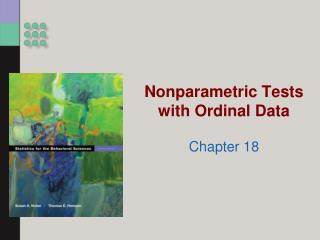# Nonparametric Tests with Ordinal Data - PowerPoint PPT PresentationDownload PresentationNonparametric Tests with Ordinal Data

Nonparametric Tests with Ordinal DataDownload Presentation## Nonparametric Tests with Ordinal Data

- - - - - - - - - - - - - - - - - - - - - - - - - - - E N D - - - - - - - - - - - - - - - - - - - - - - - - - - -
##### Presentation Transcript

1. Nonparametric Tests with Ordinal Data Chapter 18

2. The sample data for the ordinal variable, accomplishment-related national pride, are skewed. This indicates the possibility that the underlying population distribution is skewed.

3. When both variables are ordinal Quantifies association Calculating Spearman’s correlation Convert interval observations to rank-ordered observations Spearman Rank-Order Correlation Coefficient

4. The Wilcoxon Signed-Rank Test • A test for matched pairs is a nonparametric hypothesis test used when there are two groups, a within-groups design, and an ordinal dependent variable.

5. Steps for the Wilcoxon Signed-Rank Test • Step 1. Identify assumptions • Step 2. State null and research hypotheses • Step 3. Determine characteristics of comparison distribution • Step 4. Determine critical values • Step 5. Calculate the statistic • Step 6. Make a decision

6. Mann-Whitney U test • Nonparametric hypothesis test with two groups, a between-groups design, and an ordinal DV

7. Steps for the M-W U • Step 1. Identify assumptions • Step 2. State research and null hypotheses • Step 3. Determine characteristics of comparison distribution • Step 4. Determine critical values • Step 5. Calculate the statistic • Step 6. Make a decision

8. Formulae

9. Kruskal–Wallis H Test • A nonparametric hypothesis test used for more than two groups a between-groups design, and an ordinal dependent variable.

10. Steps for the K-W H Test • Step 1. Identify assumptions • Step 2. State research and null hypotheses • Step 3. Determine characteristics of comparison distribution • Step 4. Determine critical values • Step 5. Calculate the statistic • Step 6. Make a decision

11. Formulae

12. Bootstrapping • A statistical process in which the original sample data are used to represent the entire population. • We repeatedly take samples from the original sample data to form a confidence interval.